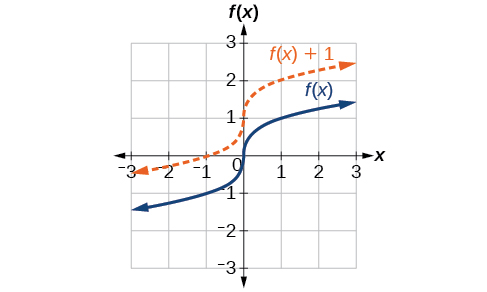# 3.5 Transformation of functions

 Page 1 / 21
In this section, you will:
• Graph functions using vertical and horizontal shifts.
• Graph functions using reflections about the $\text{\hspace{0.17em}}x\text{-axis}\text{\hspace{0.17em}}$ axis and the $\text{\hspace{0.17em}}y\text{-axis}.$
• Determine whether a function is even, odd, or neither from its graph.
• Graph functions using compressions and stretches.
• Combine transformations.

We all know that a flat mirror enables us to see an accurate image of ourselves and whatever is behind us. When we tilt the mirror, the images we see may shift horizontally or vertically. But what happens when we bend a flexible mirror? Like a carnival funhouse mirror, it presents us with a distorted image of ourselves, stretched or compressed horizontally or vertically. In a similar way, we can distort or transform mathematical functions to better adapt them to describing objects or processes in the real world. In this section, we will take a look at several kinds of transformations.

## Graphing functions using vertical and horizontal shifts

Often when given a problem, we try to model the scenario using mathematics in the form of words, tables, graphs, and equations. One method we can employ is to adapt the basic graphs of the toolkit functions to build new models for a given scenario. There are systematic ways to alter functions to construct appropriate models for the problems we are trying to solve.

## Identifying vertical shifts

One simple kind of transformation involves shifting the entire graph of a function up, down, right, or left. The simplest shift is a vertical shift , moving the graph up or down, because this transformation involves adding a positive or negative constant to the function. In other words, we add the same constant to the output value of the function regardless of the input. For a function $\text{\hspace{0.17em}}g\left(x\right)=f\left(x\right)+k,\text{\hspace{0.17em}}$ the function $\text{\hspace{0.17em}}f\left(x\right)\text{\hspace{0.17em}}$ is shifted vertically $\text{\hspace{0.17em}}k\text{\hspace{0.17em}}$ units. See [link] for an example.Vertical shift by   k = 1   of the cube root function   f ( x ) = x 3 .

To help you visualize the concept of a vertical shift, consider that $\text{\hspace{0.17em}}y=f\left(x\right).\text{\hspace{0.17em}}$ Therefore, $\text{\hspace{0.17em}}f\left(x\right)+k\text{\hspace{0.17em}}$ is equivalent to $\text{\hspace{0.17em}}y+k.\text{\hspace{0.17em}}$ Every unit of $\text{\hspace{0.17em}}y\text{\hspace{0.17em}}$ is replaced by $\text{\hspace{0.17em}}y+k,\text{\hspace{0.17em}}$ so the y -value increases or decreases depending on the value of $\text{\hspace{0.17em}}k.\text{\hspace{0.17em}}$ The result is a shift upward or downward.

## Vertical shift

Given a function $f\left(x\right),$ a new function $g\left(x\right)=f\left(x\right)+k,$ where $\text{\hspace{0.17em}}k$ is a constant, is a vertical shift    of the function $f\left(x\right).$ All the output values change by $k$ units. If $k$ is positive, the graph will shift up. If $k$ is negative, the graph will shift down.

## Adding a constant to a function

To regulate temperature in a green building, airflow vents near the roof open and close throughout the day. [link] shows the area of open vents $\text{\hspace{0.17em}}V\text{\hspace{0.17em}}$ (in square feet) throughout the day in hours after midnight, $\text{\hspace{0.17em}}t.\text{\hspace{0.17em}}$ During the summer, the facilities manager decides to try to better regulate temperature by increasing the amount of open vents by 20 square feet throughout the day and night. Sketch a graph of this new function.

We can sketch a graph of this new function by adding 20 to each of the output values of the original function. This will have the effect of shifting the graph vertically up, as shown in [link] .

Notice that in [link] , for each input value, the output value has increased by 20, so if we call the new function $\text{\hspace{0.17em}}S\left(t\right),$ we could write

$S\left(t\right)=V\left(t\right)+20$

This notation tells us that, for any value of $\text{\hspace{0.17em}}t,S\left(t\right)\text{\hspace{0.17em}}$ can be found by evaluating the function $\text{\hspace{0.17em}}V\text{\hspace{0.17em}}$ at the same input and then adding 20 to the result. This defines $\text{\hspace{0.17em}}S\text{\hspace{0.17em}}$ as a transformation of the function $\text{\hspace{0.17em}}V,\text{\hspace{0.17em}}$ in this case a vertical shift up 20 units. Notice that, with a vertical shift, the input values stay the same and only the output values change. See [link] .

 $t$ 0 8 10 17 19 24 $V\left(t\right)$ 0 0 220 220 0 0 $S\left(t\right)$ 20 20 240 240 20 20

how do I set up the problem?
what is a solution set?
Harshika
find the subring of gaussian integers?
Rofiqul
hello, I am happy to help!
Abdullahi
hi mam
Mark
find the value of 2x=32
divide by 2 on each side of the equal sign to solve for x
corri
X=16
Michael
Want to review on complex number 1.What are complex number 2.How to solve complex number problems.
Beyan
yes i wantt to review
Mark
use the y -intercept and slope to sketch the graph of the equation y=6x
how do we prove the quadratic formular
Darius
hello, if you have a question about Algebra 2. I may be able to help. I am an Algebra 2 Teacher
thank you help me with how to prove the quadratic equation
Seidu
may God blessed u for that. Please I want u to help me in sets.
Opoku
what is math number
4
Trista
x-2y+3z=-3 2x-y+z=7 -x+3y-z=6
can you teacch how to solve that🙏
Mark
Solve for the first variable in one of the equations, then substitute the result into the other equation. Point For: (6111,4111,−411)(6111,4111,-411) Equation Form: x=6111,y=4111,z=−411x=6111,y=4111,z=-411
Brenna
(61/11,41/11,−4/11)
Brenna
x=61/11 y=41/11 z=−4/11 x=61/11 y=41/11 z=-4/11
Brenna
Need help solving this problem (2/7)^-2
x+2y-z=7
Sidiki
what is the coefficient of -4×
-1
Shedrak
the operation * is x * y =x + y/ 1+(x × y) show if the operation is commutative if x × y is not equal to -1
An investment account was opened with an initial deposit of \$9,600 and earns 7.4% interest, compounded continuously. How much will the account be worth after 15 years?
lim x to infinity e^1-e^-1/log(1+x)
given eccentricity and a point find the equiationByByBy Royalle MooreByBy John GabrieliBy Laurence BailenBy OpenStaxByBy John GabrieliBy Brooke DelaneyBy OpenStaxBy Subramanian Divya